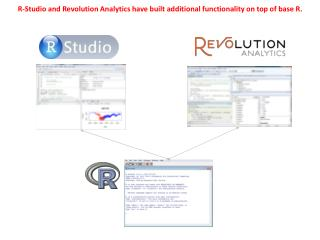# R-Studio and Revolution Analytics have built additional functionality on top of base R. - PowerPoint PPT PresentationDownload PresentationR-Studio and Revolution Analytics have built additional functionality on top of base R.

R-Studio and Revolution Analytics have built additional functionality on top of base R.
Download Presentation## R-Studio and Revolution Analytics have built additional functionality on top of base R.

- - - - - - - - - - - - - - - - - - - - - - - - - - - E N D - - - - - - - - - - - - - - - - - - - - - - - - - - -
##### Presentation Transcript

1. R-Studio and Revolution Analytics have built additional functionality on top of base R.

2. Revolution Analytics has moved onto the radar screen for predictive analytics http://www.forrester.com/pimages/rws/reprints/document/85601/oid/1-KWYFVB

3. Write Code/ Program • Input Data • Analyze • Graphics Datasets, etc. Enter Commands View Results

4. Data Structures character vector numeric vector Dataframe: d <- c(1,2,3,4)e <- c("red", "white", "red", NA)f <- c(TRUE,TRUE,TRUE,FALSE)mydata <- data.frame(d,e,f)names(mydata) <- c("ID","Color","Passed") List: w <- list(name="Fred", age=5.3) Numeric Vector: a <- c(1,2,5.3,6,-2,4) Character Vector: b <- c("one","two","three") Framework Source: Hadley Wickham Matrix: y<-matrix(1:20, nrow=5,ncol=4)

5. Actor Heights Create Vectors of Actor Names, Heights, Date of Birth, Gender 2) Combine the 4 Vectors into a DataFrame

6. Variable Types • Numeric: e.g. heights • String: e.g. names • Dates: “12-03-2013 • Factor: e.g. gender • Boolean: TRUE, FALSE

7. Creating a Character / String Vector • We use the c() function and list all values in quotations so that R knows that it is string data. • ?c  Combine Values into a Vector or List

8. Creating a Character / String Vector • Create a variable (aka object) called ActorNames: ActorNames <- c(“John", “Meryl”, “Jennifer", “Andre")

9. Class, Length, Index class(ActorNames) length(ActorNames) ActorNames

10. Creating a Numeric Vector / Variable • Create a variable called ActorHeights(inches): ActorHeights <- c(77, 66, 70, 90)

11. Creating a Date Variable • Use the as.Date() function: ActorDoB <-as.Date(c("1930-10-27", "1949-06-22", "1990-08-15", "1946-05-19“ )) • Each date has been entered as a text string (in quotations) in the appropriate format (yyyy-mm-dd). • By enclosing these data in the as.Date() function, these strings are converted to date objects.

12. Creating a Categorical / Factor Variable • Use the factor() function: ActorGender <- c(“male", “female", “female", “male“ ) class(ActorGender) ActorGender <- factor(ActorGender)

13. Vectors and DataFrames Actor.DF <- data.frame(Name=ActorNames, Height=ActorHeights, BirthDate = ActorDob, Gender=ActorGender) dim(Actor.DF)

14. Accessing Rows and Columns 1 2 3 4 Actor.DF[1,] # row 1 Actor.DF[2:3,] # rows 2,3, all columns Actor.DF[1,3] Actor.DF[,2] Actor.DF[4,3] # row 1, column 3 # row 4, column 3 # column 2

15. getwd() setwd() > getwd()  "C:/Users/johnp_000/Documents" > setwd()

16. Write / Create a File • write.table(Actors.DF, “ActorData.txt", sep="\t", row.names = TRUE) • write.csv(Actors.DF, “ActorData.csv")

17. Add New Variable: Height -> Feet, Inches Actor.DF\$Feet<- floor(Actor.DF\$Height/12) Actor.DF\$Inches <- Actor.DF\$Height - (Actor.DF\$Feet *12)

18. Sort Actor.DF[with(Actor.DF, order(-Height)), ]

19. Logical Operators / Filter Actor.DF\$Height> 68 Actor.DF\$Gender == "female" ?'[' Actor.DF[Actor.DF\$Gender == "female",] http://www.statmethods.net/management/operators.html